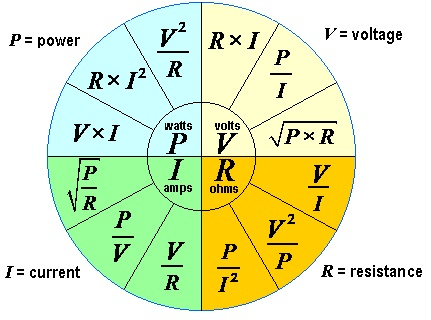# CFM Calculator

1 - Electric heat - temperature rise method:
CFM = BTU's / (ΔT x 1.08)
Input: Temp. rise(°F) ΔT , Volts & Amps

phase
formula: CFM = (Volts x Amps x 3.412) / (ΔT x 1.08)

ΔT °F
volts
amps

Enter any two parameters to get the third:
 ΔT °F BTU's Watts CFM's

2 - Velocity method:
Input square inches of duct & ft. per minute

formula: CFM = (Velocity in ft. per min.) x (area in sq. ft.)

choose duct type

Ohms Law

Volts:

Amps:

Ohms:

Watts:

Enter any two values to get the other two